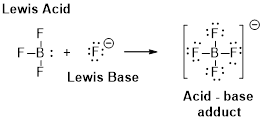Chapter 16, Problem 125SCQ

Chapter
Section
Textbook Problem

Iodine, I2, is much more soluble in an aqueous solution of potassium iodide, KI, than it is in pure water. The anion found in solution is I3−. (a) Draw an electron dot structure for I3−. (b) Write an equation for this reaction, indicating the Lewis acid and the Lewis base.

(a)

Interpretation Introduction

Interpretation:

The electron dot structure of (I3) has to be drawn.

Concept Introduction:

Valence electrons are represented by dots placed around the chemical symbol.  The valance electrons are placed around the elements (atoms) maximum electrons can fill around the atom. First fill the single electron around the atom and paired the electrons.

The valance electron for iodine atom is seven. Total valence electrons in triiodide anion are counted and electron dot structure for the anion is written. When iodine (I2) is mixed in an aqueous solution of potassium iodide (KI), an acid-base reaction occurs. Iodide anion (I) from potassium iodide acts as a Lewis base and iodine (I2) acts as a Lewis acid.

Explanation

Number of valence electrons in iodine is three. Therefore the total number of valence electrons on triiodide anion is given as:[(3×7)+1]=22.

One electron is added because the species is an anion with one negative charge

(b)

Interpretation Introduction

Interpretation:

The chemical reaction has to be written by indicating the Lewis acid and Lewis base.

Concept Introduction:

Lewis definition:

A Lewis acid is a substance that can accept and share an electron pair.

A Lewis base is a substance that can donate and share an electron pair.Still sussing out bartleby?

Check out a sample textbook solution.

See a sample solution

The Solution to Your Study Problems

Bartleby provides explanations to thousands of textbook problems written by our experts, many with advanced degrees!

Get Started